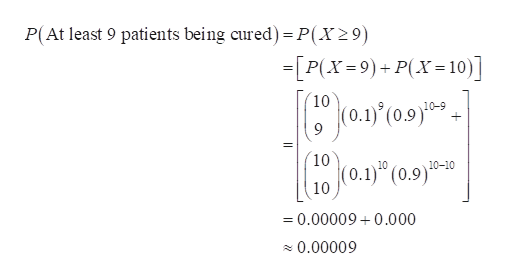# Assume that every patient with a particular type of disease has probability 0.1 of being cured within a week, if the patient is given no treatment for the disease.  Ten patients with that type of disease are given a new type of drug. After one week 9 out of 10 patients are cured.  What is the probability of at least 9 patients being cured if the drug is assumed to have No curative effects?

Question

Assume that every patient with a particular type of disease has probability 0.1 of being cured within a week, if the patient is given no treatment for the disease.  Ten patients with that type of disease are given a new type of drug. After one week 9 out of 10 patients are cured.  What is the probability of at least 9 patients being cured if the drug is assumed to have No curative effects?

check_circleExpert Solution
Step 1

Consider X as the number of successes (patients being cured) out of the n = 10 trials (patients). That is, random variable X denote the number of patients being cured.

Thus, X has a Binomial distribution with parameters n = 10 and p = 0.1. Since X is a discrete random variable, X has a Binomial pmf (probability mass function).

The pmf of the binomial random variable with parameters n = 10 and p = 0.1 is given as follows:help_outlineImage Transcriptionclosepqx0,1n,0 < p< 1;q=1-p -х f (x) |0. Here otherwise 10 10-x (0.1) (1-0.1) 0,1....10 f(x)= otherwise [0. That is 10 10-x |(0.1) (0.9) 0,1....10 f (x) (0, otherwise fullscreen
Step 2

The probability that at least 9 patients being cured if the drug is assumed to have no curative effects is same as the probability that 9 or more patients being cured. That is, the required probabi...help_outlineImage TranscriptioncloseP(At least 9 patients being cured) = P(X29) =[P(X 9)+P(X= 10) - 10 10-9 + 10 10 10-10 (0.9) 10 =0.00009 0.000 0.00009 fullscreen

### Want to see the full answer?

See Solution

#### Want to see this answer and more?

Solutions are written by subject experts who are available 24/7. Questions are typically answered within 1 hour*

See Solution
*Response times may vary by subject and question
Tagged in

### Statistics